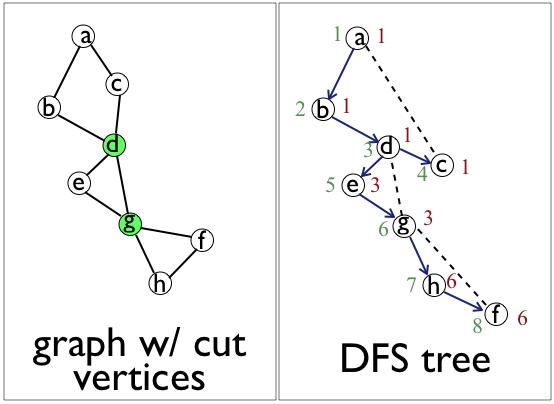CutVerticesByDFS

ClassS04CS141 | recent changes | Preferences

Difference (from prior major revision) (no other diffs)

Removed: 1d0

Changed: 16c15Changed: 108c107
 * CormenEtal? problem 23-2 (Articulation points)
 * CormenEtal? problem 23-2 (Articulation points)

Problem statement, introduction to algorithm

A cut vertex (in a connected, undirected graph) is a vertex whose removal disconnects the graph.

A simple algorithm for identifyiing cut vertices:

1. For each vertex w, remove w from the graph and see if the graph becomes disconnected, using DFS or BFS.

Time for this one is O(n(n+m)), where n=#vertices, m=#edges.

To get a faster algorithm, consider the DFS tree of the graph. In particular, consider the back edges:

The graph is on the left, the cut vertices are in green.

The DFS tree is on the right, the back edges are the dotted edges.

Claim: A non-root vertex U is a cut vertex if and only if one of its children W in the DFS tree has the following property:

• there is no descendant of W with a back edge reaching above U.

To determine whether this property holds for a vertex, define:

• The dfs-number of each vertex by labelling the vertices 1,2,...,n in the order in which DFS first encounters them. Above, the dfs-number of each vertex is shown in green next to the vertex.
• For each vertex v, define low[v] = min { dfs-number[v] , min { dfs-number[w] : (u,w) is a back edge for some descendant u of v }}. That is, low[v] is the smallest dfs-number reachable by taking tree edges down from v and then at most one back edge up.

Claim: A non-root vertex U in the DFS tree is a cut vertex if and only if one of its children W in the DFS tree has:

• low[W] >= dfs-number[U].

(Prove this later.)

Claim: A root vertex is a cut vertex if and only if it has two or more children in the DFS tree (using just tree edges).

(Prove this later.)

Claim: the low[] numbers satisfy the following recurrence relation:

• low[v] = min { dfs-number[v], min {dfs-number[w] : (v,w) is a back edge}, min {low[w] : w is a child of v in the dfs-tree } }

(Prove this later.)

To finish, the low[] numbers can be computed bottom-up using the DFS tree using the recurrence. This computation takes O(n+m) time.

To summarize, here is the outline of an O(n+m)-time algorithm for identifying cut vertices:

1. Do a DFS on the graph.
2. Compute the dfs number for each vertex.
3. Compute the low number for each vertex.
4. The cut vertices are the non-root vertices U having a child V such that low[V] >= dfs-number[U], plus the root vertex, if the root vertex has degree two or more in the dfs tree.

Proving the claims

The argument for correctness of the algorithm rested on the following three claims:

1. A non-root vertex U is a cut vertex if and only if one of its children W in the DFS tree has the following property:

there is no descendant of W with a back edge reaching above U.

2. The root vertex of the dfs tree is a cut vertex if and only if it has two or more children in the DFS tree (using just tree edges).

3. The low numbers satisfy the following recurrence relation:

low[v] = min { dfs-number[v], min {dfs-number[w] : (v,w) is a back edge}, min {low[w] : w is a child of v in the dfs-tree } }

To verify the algorithm, we need to consider carefully whether the claims are true. In particular, are we convinced the claims will hold when the algorithms are run on any input.

Next we will do this, partly, to give an idea of what's involved.

For the first claim to be true, it must be that, for any input,

1A. If a non-root vertex U is a cut vertex, then it will have a child W in the DFS tree such that no descendant of W has a back edge above U.

and

1B. If a non-root vertex U has such a child, then it is a cut vertex.

The second part (1B) is easy to argue for. If U has a child W, with no descendant of W having a back edge going to a vertex above U, then removing W disconnects the child from the parent of W.

The first part (1A) is a little less obvious. We tried to come up with a line-by-line justification:

1. Suppose U is a cut vertex.
2. Removing U from the graph separates the graph into at least two components.
3. The root R of the DFS tree is in one of these components, say, C.
4. There is at least one other component, say, C'.
5. Let W be the first vertex in C' encountered by the DFS.
6. Then consider where W has to be in the DFS tree...
• Since all paths from R to W go through U, W has to be a descendant of U in the DFS tree.
• Since W is the first vertex in C' encountered by the DFS, W has to be the immediate child of U in the DFS tree.
• Since all paths from R to W go through U, there cannot be a descendant of W that has a back edge going above U.

This seems to be a convincing and general argument that (1A) is true. If we accept this argument, then we believe (1A) is true. If we accept that (1B) is also true, then we believe claim (1).

Note that to really convince ourselves that the algorithm is correct, we need to verify also that claims (2) and (3) are correct. We leave these as exercises (for claim (3), see DynamicProgramming).

References

• CormenEtal? problem 23-2 (Articulation points)

ClassS04CS141 | recent changes | Preferences